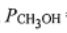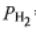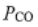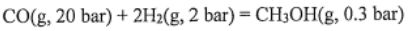110 年 - 110 高雄科技大學_碩士班招生考試_化學工程與材料工程系：物理化學(乙組)#104402

【非選題】
1.

1. A mixture of CO(g), H2(g), and CH3OH(g) at 500 K with= 0.3 bar,=2 bar, and= 20 bar is passed over a catalyst.【題組】

(a) The standard Gibbs energy of reaction is 21.21. What is the value of the Gibbs energy of reaction in【非選題】
2.【題組】(b) Can more methanol be formed? Why?
Note: In(cd) = In(c) + In(d),= nIn(m)

【非選題】
3.

2. (a) Show that= 0 for an ideal gas, whereand P are molar enthalpy and pressure,
respectively. You can start from the equation belowwhereis molar entropy,is molar volume, and T is temperature

【非選題】
4.(b) A piece of stretched rubber exerts a force of 5 N. How much work (in J) has to be done on the rubber to stretch it one centimeter?

【非選題】
5.

3. The density of liquid water at 300 K is. Activity is represented by a. What is the value of In(a) of liquid water at 80 bar at 300 K?

Note: In
(D) = Natural logarithm of D,= m,【非選題】
6.4. A and B represent different substances. A and B cannot occur chemical reaction. Assuming that A and B are ideal gases, what is the entropy (in J) of mixing of 2 mol A with 3 mol of B at 300 K?
Note: In(cd) = In(c) + In(d),= nIn(m)

【非選題】
7.5.ine burns a stoichiometric mixture of fuel (liquid hydrogen) in oxidant (liq The combustion chamber is cylindrical, 1.2 m long and 80 cm in diameter, and the combustion process produces 306 kg s' of exhaust gases. 2H2(I) + O2(I) = 2H2O(g). The reactor volume and me in which reaction takes place are identical. If combustion is complete, value of the rate of reaction of oxygen in kgmol m3 sl?
Note:【非選題】
8.6. The galvanic cell has standard electromotive force E° of 0.1 V at 25℃. K is the equilibrium constant. Assumning the charge number for the reaction is unity, what is the value of In(K) of the reaction that occur in the cell?
Note: In
(D) = Natural logarithm of D,= m

懸賞詳解

國一生物下第一次

【題組六】 豌豆莖的高矮由一對等位基因所控制，高莖為顯性，以 T 代表，矮莖為隱性，以 t 代表。現將兩 株豌豆進行交配產生子代，其過程如附圖(�...